Courses

# Sample Paper 1 with Solution - Science, Class 9 Class 9 Notes | EduRev

## Class 9 : Sample Paper 1 with Solution - Science, Class 9 Class 9 Notes | EduRev

The document Sample Paper 1 with Solution - Science, Class 9 Class 9 Notes | EduRev is a part of Class 9 category.
All you need of Class 9 at this link: Class 9

Section A

Ques 1: What is intercropping? (1)
Ans:
The practice of growing two or more crops simultaneously in the same field in a definite row pattern is called intercropping.

Ques 2: Define biogeochemical cycle. (1)
Ans:
Movement of nutrient elements through the living and non-living components of the biosphere is called a biogeochemical cycle.

Section B

Ques 3: List any three human activities which could lead to an increase in carbon dioxide content of air.
OR
Explain the role of the Sun in the formation of soil.  (2)
Ans:
Human activities which could lead to an increase in carbon dioxide content of air:
(a) Burning of fossil fuels such as coal and petroleum
(b) Deforestation
(c) Burning associated with agricultural practices
OR
Role of the Sun in the formation of soil:
· The Sun plays an important role in weathering of rocks for soil formation.
· It heats up the rocks during the day. Heating causes the rocks to expand.
· During the night, the rocks cool down and contract. Different parts of a rock expand and contract at different rates resulting in its cracking and breaking up into smaller pieces or fragments.

Ques 4: What is the significance of electronic configuration of an atom? (2)
Ans:
The electronic configuration of an atom plays an important role in determining the chemical behaviour of an element.
· When the atom of an element has a completely filled outermost shell, the element will be chemically unreactive.
· When the atom of an element has less than 8 electrons in its outermost shell, the element will be reactive.

Ques 5: Which of the following situations indicates Newton’s first law of motion:
(a) A ball thrown upwards reaches a point and then starts falling.
(b) Passengers of the car feel a jerk when the driver applies emergency brakes.
(c) A gunman moving backwards as soon as the gun shoots a bullet.
(d) Formation of ripples on the surface of the lake when a stone is thrown into it. (2)
Ans:
Newton’s first law of motion is the law of inertia which states that a body at rest will continue to stay at rest and a body in motion will continue to be in the state of motion until an external force is applied to it.
Therefore, case (ii) indicates Newton’s first law.
Newton’s first law of motion is the law of inertia which states that a body at rest will continue to stay at rest and a body in motion will continue to be in the state of motion until an external force is applied to it.
Therefore, case (ii) indicates Newton’s first law.

Section C

Ques 6: State the observations from the α-particle scattering experiment which led Rutherford to make the following conclusions: (3)
a. Most space in an atom is empty.
b. Entire mass of an atom is concentrated at its centre.
c. Centre is positively charged.
Ans:
(a) Most rays passed through the thin gold foil undeviated.
(b) Very few rays returned in the same path.
(c) Some rays deflected through larger angles.

Ques 7: Explain any three methods of weed control. (3)
OR
What is green manuring? List two commonly used green manure crops and name two macronutrients provided by green manure.
Ans:
Methods of weed control:
(a) Mechanical method: It involves methods such as uprooting weeds manually, weeding with a trowel, hand hoeing, ploughing and burning.
(b) Chemical method: It involves the use of chemical weed killers called herbicides or weedicides to kill or destroy weeds.
(c) Biological method: It involves the deliberate use of insects or some other organisms which consume and specifically destroy weed plants.
OR
Green manuring is the practice of ploughing green plants into the soil for improving its fertility. Green manure provides organic matter and nutrients such as nitrogen and phosphorus to the soil.
Commonly used green manure crops: Guar and Sunn hemp
Macronutrients provided by green manure: Nitrogen and phosphorus

Ques 8: Give reasons: (3)
(a) A gas completely fills the vessel in which it is kept.
(b) A gas exerts pressure on the walls of the container.
(c) A wooden table should be called a solid.
Ans:
(a) The force of attraction between particles of a gas is negligible or less. So, the particles freely move/flow in all possible directions, as a result the gas completely fills the vessel in which it is kept.
(b) Freely moving particles of a gas hit the walls of its container continuously and randomly. Hence, such random and erratic motion of gas particles exerts pressure on the walls of the container.
(c) Particles of a wooden table are rigid and have a fixed location. They also possess a definite shape and volume. Because of these properties, we should call a wooden table a solid substance.

Ques 9: Define and explain the differences between (3)
(a) Distance and displacement
(b) Speed and velocity
Ans:
(i) Distance: It is the actual length covered by a body during the course of motion.
As the distance only indicates the magnitude of the length covered without any element of the direction of the motion, it is a scalar quantity. The distance covered cannot be negative.
(ii) Displacement: Displacement is the change in position made by the body during motion. Direction of the movement along with the magnitude of the change of position determines the value of displacement; therefore, it is a vector quantity.

Ques 10: Although a stone and the Earth attract each other with equal force, why do we observe that only the stone falls towards the Earth but not the Earth rising towards the stone? Explain.(3)
Ans:
Force (F) acting between the Earth (mass M) and the stone of mass (m) separated by the distance (r) by the universal law of gravitation is given by the equation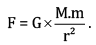The mass of stone is negligible (too less) when compared to the mass of the Earth which is 6 * 1024 kg. Thus, the stone falls on the Earth and the Earth does not rise towards the Sun.

Ques 11:A wooden cuboid has a mass of 8 kg. The length, breadth and height of this wooden cuboid are 100 cm, 50 cm and 20 cm, respectively. Find the pressure on the floors on which this block is kept. (3)
OR
Calculate the force of gravitation due to the Earth on a ball of 2 kg mass lying on the floor. Consider the mass of the Earth = 6 * 1024 kg, radius of the Earth = 6400 km and universal gravitation constant G = 6.7 * 10−11 Nm2/kg2.  (3)
Ans:
The pressure is the ratio of force (F) exerted by a body to the area (A) upon which the body is exerting the force. Also, weight (W) is the force exerted by a body due to the Earth’s gravitational pull, i.e. F = W.
W = mg
Acceleration due to gravity (g) = 10 m/s2
∴ W  = 8 * 10 = 80N
Pressure (P) =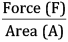Area of the surface of the cuboid (A)= l * b
Assuming the surface of 50 * 20 cm to be in contact with the floor.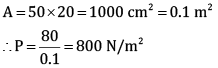Pressure exerted by the block on the floor is 800 N/m2.
OR
The force of gravitation is calculated by using the equation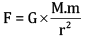Gravitation constant G = 6.7  * 10-11 Nm2/kg2
Mass of the Earth  = 6 * 1024 kg
Mass of ball = 2 kg
As the ball is lying on the floor, the distance between the ball and the Earth is equal to the radius of the Earth. Substituting all the relevant values in the above equation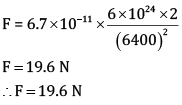Ques 12: A silver ornament of mass ‘m’ gram was polished with gold equivalent to 1% of the mass of silver. Calculate the ratio of the number of atoms of gold and silver in the ornament. (3)
OR
Write the chemical formula of the following using the criss-cross method: (3)
a. Ammonium sulphate
b. Magnesium bicarbonate
c. Barium nitrate
Ans:
Mass of silver in the ornament = m gram
Mass of gold in the ornament =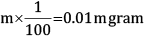108 g of Ag contains 6.022 * 1023 atoms
m gram of Ag contains(6.022 * 1023 /108) * m /100 atoms ratio of number of atoms of gold and silver = Au: Ag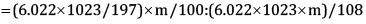= 108:19700
=1:182.41
OR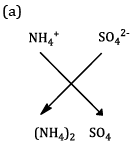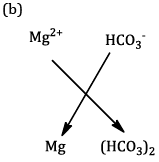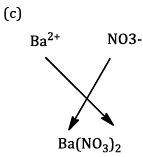Ques 13: The doctor diagnosed that Lata has lost the power of fighting any infection. (3)
(a) Name the disease Lata is suffering from.
(b) Name the pathogen responsible for the cause of the disease.
(c) Mention any two modes of transmission of the disease from one person to another.
Ans:
(a) AIDS (acquired immunodeficiency syndrome)
(b) Retrovirus called HIV (human immunodeficiency virus)
(c) Modes of transmission of the disease:
(i) Unprotected sexual contact with an HIV-infected person
(ii) Transfusion of blood contaminated with HIV

Ques 14: Why does the skin of your fingers shrink when you wash clothes for a long time? (3)
Ans:
(a) Clothes are washed with soap or detergent solution. This solution is hypertonic because it contains low water concentration as compared to the osmotic concentration of our skin cells.
(b) Therefore, when skin cells come in contact with the detergent solution, they begin to lose more water by exosmosis after some time.
(c) As a result, the skin over the fingers shrinks while washing clothes for a long time.

Ques 15: We know that carbon dioxide is essential for photosynthesis in plants. Yet, why do we consider it a pollutant? (3)
Ans:
(a) Carbon dioxide is required by plants to make food by photosynthesis.
(b) It is also a greenhouse gas which traps the heat reflected by the Earth’s surface and keeps the atmosphere warm.
(c) However, when the concentration of CO2 rises above normal levels, it traps more heat resulting in heating of the Earth’s atmosphere and an increase in the Earth’s temperature causing global warming.
(d) Global warming is dangerous because it tends to melt polar ice and glaciers on mountains. This causes a rise in the water level of oceans and submerges several coastal areas and islands.
(e) Therefore, although carbon dioxide is essential for plants, it can act as a pollutant in case of excess concentrations.

Section D

Ques 16: (a) Define the terms and give one example of each: (5)
(i) Bilateral symmetry
(ii) Coelom
(iii) Diploblastic
(b) Identify the group of animals with
(i) Spiny body and radial body symmetry
(ii) Four pairs of jointed legs and no wings

Ans: (a) (i) Bilateral symmetry: The type of body symmetry in which two sides of the body are mirror images of one another is called bilateral symmetry.
Example: Earthworm
(ii) Coelom: Body cavity lined with an epithelium derived from the mesoderm is called coelom.
Example: Spider
(iii) Diploblastic: Animals which have two germ layers—outer ectoderm and inner endoderm—in the embryo are said to be diploblastic.
Example: Hydra
(b)
(i) Echinodermata
(ii) Arachnida

Ques 17: (a) Work done by a force is given by the equation W = F cos ϴ * s. Determine and explain the effect of work done due to a gradually increasing angle ‘ϴ’.
(b) A car is being driven by a force of 5 * 1010 N.  It takes two minutes with the speed of 10 m/s for this car to reach the river side. Calculate the work done.
OR
(a) Explain the work done by a person in the following conditions:
i. When he is standing at a place holding a suitcase in his hand.
ii. When he is moving, holding the suitcase in his hand.
(b) A certain household has consumed 250 unit of energy during a month. How much energy is consumed in joules?
Ans: (A) Work (W) done by a force (F) is given by
W = F * s Cosθ
The range of angle 'θ ' in simple trigonometry is from 00 to 900
Cos0=1 and Cos90=0
Therefore, as the angle between directon of force and direction of motion increases, the work done by a force reduces.
(b) Work (W) = Force (F) Displacement (s) ...(1)
Force (F)= 5 * 1010 N
In this case of motion, the displacement of the car is equal to the distance travelled by the car.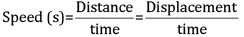Speed (s) = 10 m/s
Time of motion (t) = 2 min = 120 secs
∴ Displacement (s) = 1200 m
Substituting the above value of displacement in equation (1)
Work done =Therefore, work done by the car to reach the river side is 6 *1013 joule.
OR
(a)
i. When the person is standing at a place holding a suitcase, there is no change in the position of the man or the suitcase.
Therefore, displacement (s) = 0
W = F × s = F × 0 = 0
ii. When the person is moving holding the suitcase in his hand, he applies force in the upward direction and the displacement of the suitcase is in the forward direction, i.e. perpendicular to the direction of the force applied.
Therefore, θ = 90°
Since W = F × s cosθ
W = F × s cos90˚ = 0
Hence, work done on the suitcase is zero.
(b) Energy consumed = 250 units = 250 kWh
1 kWh = 3.6 × 106 J
∴ Energy consumed = 250 × 3.6 × 106 J = 9 x 10J

Ques 18: Distinguish between true solution and colloid. (5)
OR
(a) What is an octet? How does an element reach an octet state? (3)
(b) Draw a schematic atomic structure of magnesium and phosphorus (Number of protons of magnesium = 12, phosphorus = 15). (2)
Ans:

 True Solution Colloid 1. A true solution is a homogeneous mixture of two or more substances. 1. A colloidal solution is a heterogeneous mixture of two substances. 2. Size of the particles is less than one nanometre. 2. Range of particle size is from 1 to 100 nanometre. 3. It is always transparent. 3. It is translucent. 4. Particles cannot be seen even with a microscope. 4. Particles of a colloidal solution can be seen with a microscope. 5. It does not show Tyndall effect. 5. It shows Tyndall effect.

OR
(a) An atom has an octet when it has 8 electrons in the outermost shell. An element can attain its octet by losing, gaining or sharing electrons.
(b) Magnesium atom: 12 n, 12 p, 12 e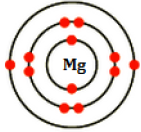Phosphorus atom: 16 n, 15 p, 15 e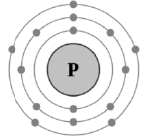Ques 19: (a) A man weighs 300 N on the surface of the Earth. If he were taken to the Moon, his weight would be 50 N. Calculate the mass of this man on the Moon (g = 10 m/s2). (3)
(b) A man hears an echo of thunder 2 seconds after lightning strikes. Calculate the distance of lightning from the man (Speed of sound in air = 330 m/s).  (2)
Ans:
(a) Weight of man on the Earth (WEarth) = 300 N
acceleration due to gravity (gEarth )= 10 m/s2
Weight α acceleration due to gravity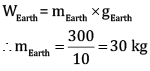Weight of man on the moon (Wmoon) = 50 N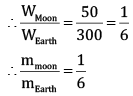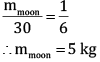Therefore, the mass of the man on the Moon is 5 kg.
(b) We know that
Speed of sound in air (v)= Distance/time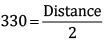∴ Distance = 660 m
Therefore, the distance between the man and the point of lightning is 660 m.

Ques 20: (a) How does cork act as a protective tissue? (5)
(b) Observe the figure carefully and label the parts marked A and B.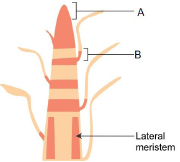Or
(a) Write the location and function of collenchyma tissue.
(b) Draw a diagram of collenchyma tissue and label any four of its parts.
(c) Identify the region of the stem marked 'A' in the diagram below and the type of simple permanent tissue found in this region.
(d) Mention any two characteristic features of the cells found in this tissue.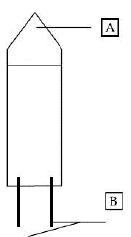Ans:
(a)
(i) Cork cells are dead and compactly arranged without intercellular spaces.
(ii) Cell walls are coated with an organic substance called suberin which makes these cells impermeable to water and gases.
(iii) As a result, cork cells prevent desiccation, infection and mechanical injury to the plant body.
(iv) Cork is light and does not catch fire easily because of which it is used as an insulator, shock absorber, in linoleum and in making sports goods.
(b) A – Apical meristem; B – Intercalary meristem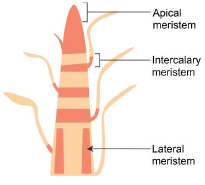OR
(a) Collenchyma tissue:
Location: In the leaf stalk and below the epidermis.
Function: It allows easy bending in various parts of a plant without breaking (i.e. it provides flexibility to plants).
(b) Structure of collenchyma tissue: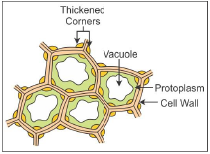(c) 'A' is pith. The given diagram is of parenchyma tissue.
(d) Characteristic features of parenchyma tissue:
. Cells are thin walled and are loosely packed.
· These are living cells and help in storage.

Ques 21: What happens when sugar is dissolved in water? Where does the sugar go? What information do you get about the nature of matter from the dissolution of sugar in water?(5)
Ans:
When sugar is dissolved in water, its crystals break down into tiny particles. The sugar particles go into the spaces between the particles of water and mix with them to form sugar solution. Sugar particles occupy the space between water particles.
From the dissolution of sugar in water, we can infer
(i) Matter (consisting of sugar and water) is made of small particles.
(ii) Particles of matter have spaces between them.

Section E

Ques 22: Observe the figures A, B and C. (2)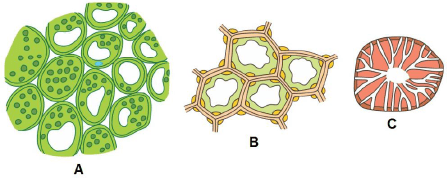(a) Which of these tissues provide both mechanical strength and flexibility?
(b) Which of these tissues can be modified to form air cavities in aquatic plants?
OR
Deepa was asked to draw the structure of a neuron as observed under a microscope. Observe the figure drawn by her and answer the questions based on it.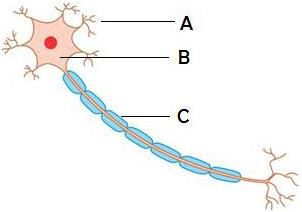(a) Identify A, B and C.
(b) What is the function of part A?
Ans:
A – Parenchyma, B – Collenchyma, C – Sclerenchyma
(a) Tissue B or collenchyma provides both mechanical strength and flexibility.
(b) Tissue A or parenchyma can be modified to aerenchyma with air cavities in aquatic plants.
OR
(a) A → Dendrites
B → Cyton
C → Axon
(b) Part A or dendrites receive signals from other neurons, process these signals and transfer the information to the soma of the neuron.

Ques 23: Rishi observed a permanent slide of Spirogyra. (2)(a) Which cell organelles can be clearly seen and labelled from this slide?
(b) To which group of plants does Spirogyra belong?
Ans:
(c) A permanent slide of Spirogyra shows a nucleus, ribbon-shaped chloroplast, cell wall and pyrenoids.
(d) Spirogyra belongs to Division Thallophyta.

Ques 24: Identify two clear and transparent solutions from the following:
(a) Milk and water
(b) Sugar and water
(c) Starch and water
(d) Alum in water
(e) Egg albumin and water
(f) Chalk powder and water (2)
OR
In test tube one, 5 g of zinc dust was taken. In test tube two, 5 g of granulated zinc was taken. 10 ml of dilute sulphuric acid was added to each test tube.  Identify the gas evolved during the reaction and comment on the rate of evolution of gas in both test tubes.

Ans: Two clear and transparent solutions are (b) sugar and water, (d) alum and water.
OR
Hydrogen gas will evolve during the reaction.
The rate of reaction is more in test tube one than in test tube two.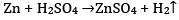Ques 25: What happens when solutions of sodium sulphate and barium chloride are mixed? Give the reaction.  (2)
Ans: When solutions of sodium sulphate and barium chloride are mixed, a white-coloured precipitate of barium sulphate is formed along with sodium chloride solution.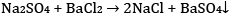Ques 26: While performing an experiment based on Archimedes principle, the following changes were observed. What will be the volume of the stone immersed in the liquid? (2)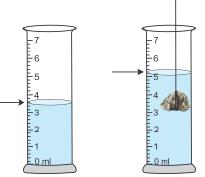(a) 3.6 ml
(b) 1.5 ml
(c) 2 ml
(d) 5.1 ml
Ans:
According to the Archimedes principle, the volume of the stone is equal to the volume of liquid displaced due to the immersion of that stone. The volume of liquid displaced can be found by calculating the difference between the original volume of liquid before immersion of the stone and the changed volume of the liquid after immersion of the stone. So,  5.1 − 3.6 = 1.5 ml

Ques 27: A student has set up a slinky on a smooth table top in the manner shown below. How can he produce transverse waves in the slinky by moving its free end Q? (2)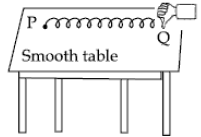(a) At an angle of 45° with the table top
(b) Backward and forward along the length of the slinky
(c) Up and down
(d) Left and right
OR
(a) If we place the porous surface at the back of the hollow tubes in the experimental setup of the reflection of sound, then
(A)Sound will be heard with greater intensity than the incident sound.
(B) Sound will be heard with lesser intensity than the incident sound.
(C) Reflection of sound does not take place.
(D)Reflected sound will remain the same as incident sound.
(b) Explain why.
Ans:
A student can produce transverse waves by vibrating the freely moving end ‘Q’ of the slinky in the ‘up and down’ direction.
OR
(a) No reflection of sound takes place.
(b) No reflection of sound will take place from the porous surface as it absorbs the sound.

Offer running on EduRev: Apply code STAYHOME200 to get INR 200 off on our premium plan EduRev Infinity!

,

,

,

,

,

,

,

,

,

,

,

,

,

,

,

,

,

,

,

,

,

,

,

,

;